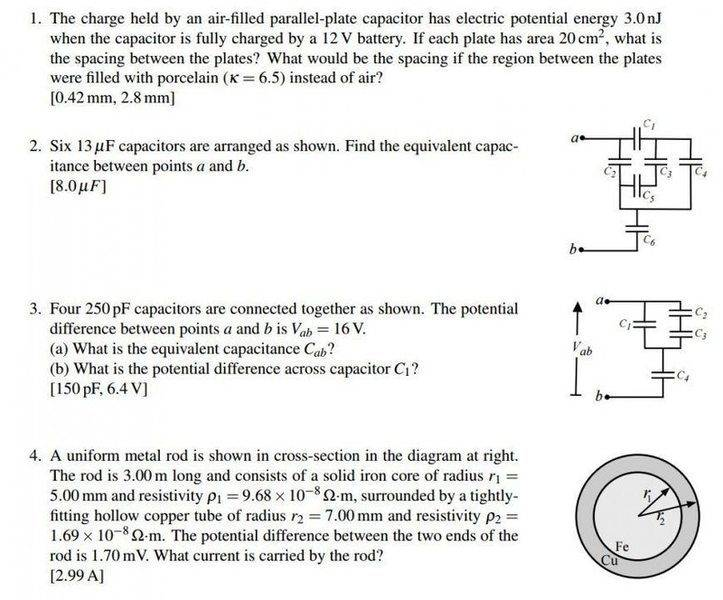# Help with capacitance

• gamma-guy
In summary, the student is asking for help with equations for Q1, Q4 and Q2. He is working on solving Q1 and Q4 but needs help with Q2. He has found that if he does C23 series and parallel, and then series with C4, he gets the right answer. He is unsure about what he is doing wrong for Q3.

#### gamma-guy

reminder: members should give each h/w question its own thread

## Homework Statement

Ive got these questions and I am not quite sure where to start. if someone could point me to the equations to use for Q1 and Q4, id appreciate it. As for Q2 and Q3, I need help with how to determine if its in series or parallel and how to properly add them.## Homework Equations

q=CV
Here is a datasheet I was told would be helpful.

## The Attempt at a Solution

so I got 3a) by doing C23 series, = (1/250 +1/250)-1 = 125uF. Then parallel with C1 = 125 + 250 = 375uF. Then series with C4 = (1/375+1/250)-1 and got 125uF.

For b) I thought of doing q= CV = 150e-12*16V = 2.4e-9. Then because capacitance is the same, V = q/C = 2.4e-9/250e-12. But that gives me 9.6V. I am supposed to get 6.4V. What am I doing wrong?[/B]

Last edited by a moderator:
Your answer for 3a looks correct. For 3b check if you calculated the voltage on C1 or C4. I note that 16-9.6=6.4

As for equations, look up the one for the energy stored on a capacitor and the one for the capacitance in terms of plate area and separation.

If you can do Q3a you can do Q2.

gamma-guy said:
so I got 3a) by doing C23 series, = (1/250 +1/250)-1 = 125uF. Then parallel with C1 = 125 + 250 = 375uF. Then series with C4 = (1/375+1/250)-1 and got 125uF.
Your analysis is correct but check the calculations for C4 again. The answer is 150pF as given.
gamma-guy said:
. Then because capacitance is the same, V = q/C = 2.4e-9/250e-12.
What do you mean by " the capacitance is same"? There will be division of charge between C1 and C23. Voltage across C1, C23 and C123 is same.

Thanks guys, ill look over it.

Any help with Q4?

gamma-guy said:
Thanks guys, ill look over it.

Any help with Q4?
Can you make an attempt? What are your thoughts on how you might approach the problem? What would you do if the rod was just a solid cylinder of iron or copper?